• Slides: 31Solving Quadratic Equations What is the definition of a solution to a quadratic equation? 0 A solution is the value of x when y = 0. What are other terms for the solutions to a quadratic equation? x-intercepts (in some cases)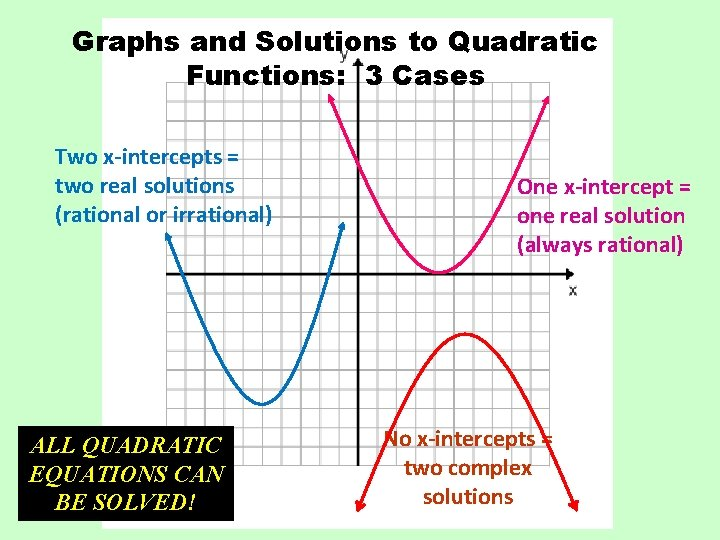Graphs and Solutions to Quadratic Functions: 3 Cases Two x-intercepts = two real solutions (rational or irrational) ALL QUADRATIC EQUATIONS CAN BE SOLVED! One x-intercept = one real solution (always rational) No x-intercepts = two complex solutions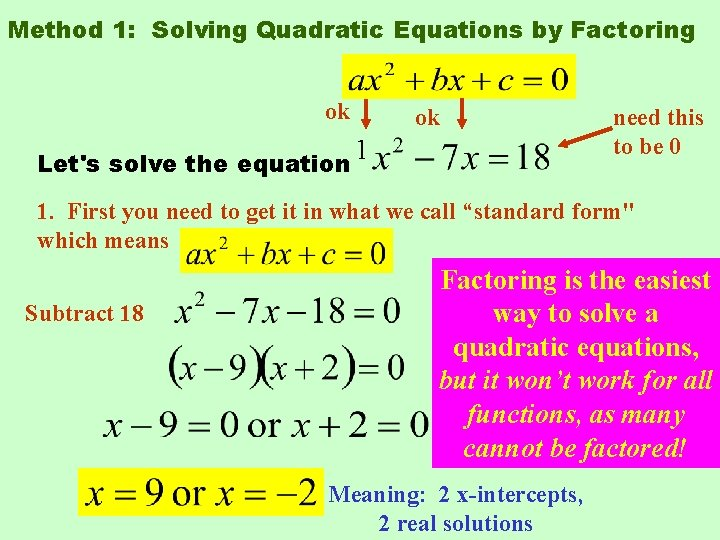Method 1: Solving Quadratic Equations by Factoring ok ok 1 Let's solve the equation need this to be 0 1. First you need to get it in what we call “standard form" which means Subtract 18 Factoring is the easiest way to solve a quadratic equations, 2. Now let's factor left hand but itthe won’t workside for all functions, many= 0 3. Now set eachasfactor beeach factored! andcannot solve for answer. Meaning: 2 x-intercepts, 2 real solutions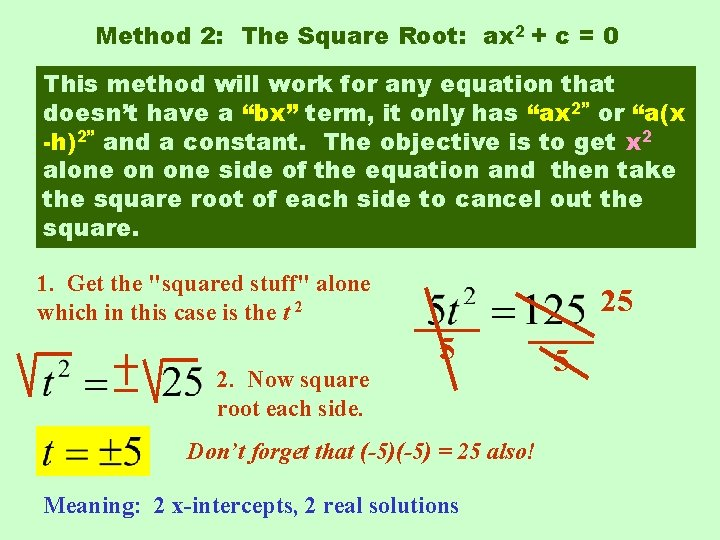Method 2: The Square Root: ax 2 + c = 0 This method will work for any equation that doesn’t have a “bx” term, it only has “ax 2” or “a(x -h)2” and a constant. The objective is to get x 2 alone on one side of the equation and then take the square root of each side to cancel out the square. 1. Get the "squared stuff" alone which in this case is the t 2 2. Now square root each side. 25 5 Don’t forget that (-5) = 25 also! Meaning: 2 x-intercepts, 2 real solutions 5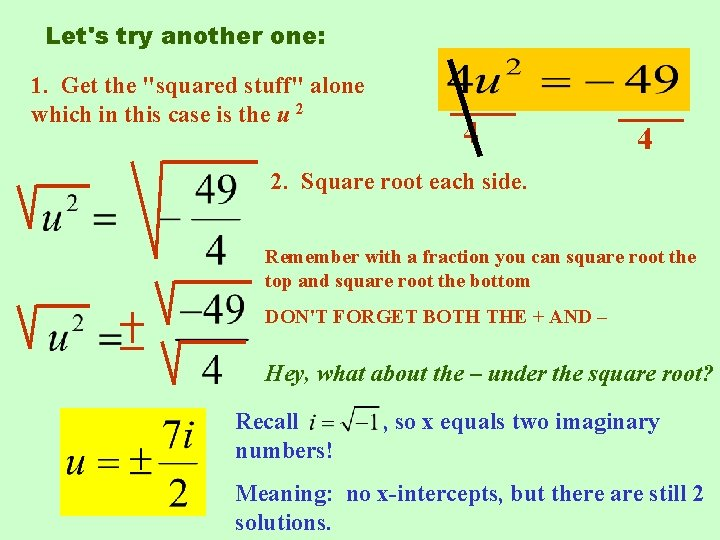Let's try another one: 1. Get the "squared stuff" alone which in this case is the u 2 4 4 2. Square root each side. Remember with a fraction you can square root the top and square root the bottom DON'T FORGET BOTH THE + AND – Hey, what about the – under the square root? Recall numbers! , so x equals two imaginary Meaning: no x-intercepts, but there are still 2 solutions.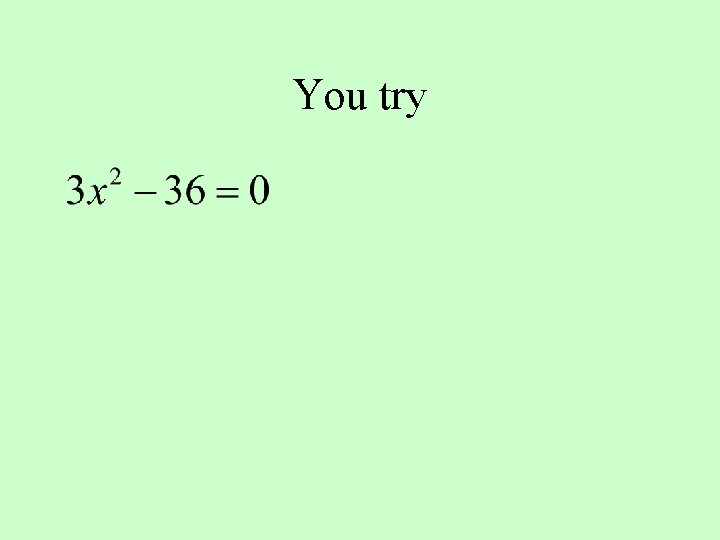You try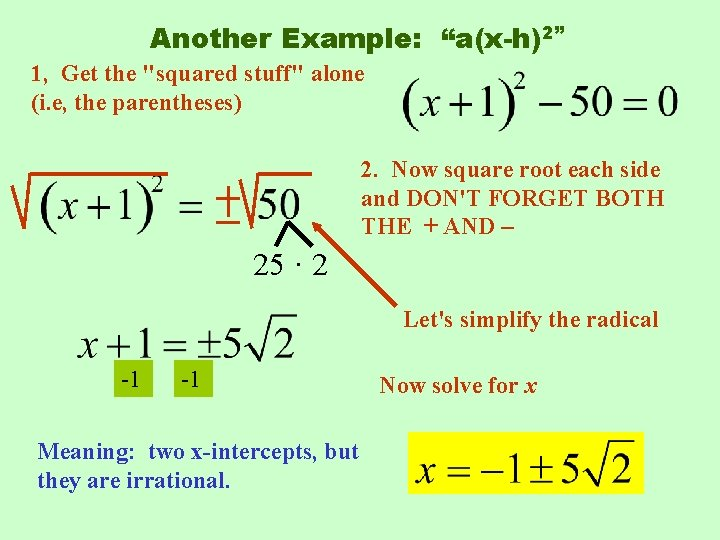Another Example: “a(x-h)2” 1, Get the "squared stuff" alone (i. e, the parentheses) 2. Now square root each side and DON'T FORGET BOTH THE + AND – 25 · 2 Let's simplify the radical -1 -1 Meaning: two x-intercepts, but they are irrational. Now solve for xPerfect Square Trinomials: What’s the pattern? Add how much? c=? Factored form 1 4 9 16 “add half of b squared” To complete the square and make a perfect square trinomial, _________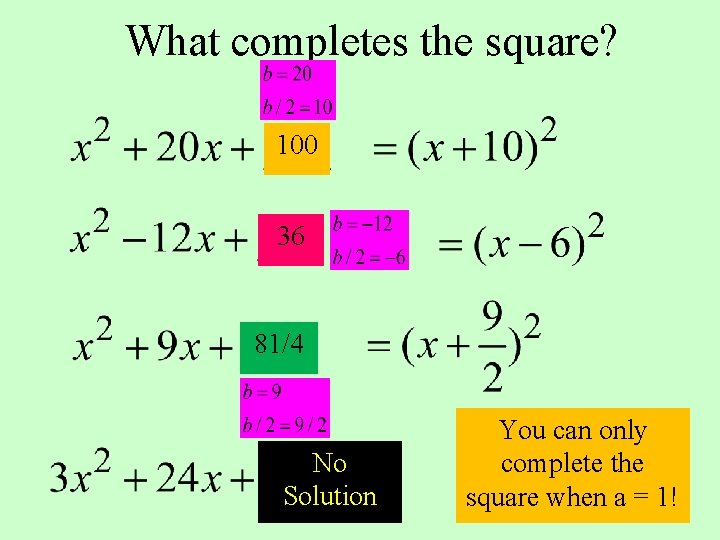What completes the square? 100 36 81/4 No Solution You can only complete the square when a = 1!Method 4: The Quadratic Formula is a formula that can solve any quadratic, but it is best used for equations that cannot be factored or when completing the square requires the use of fractions. It is the most complicated method of the four methods. Do you want to see where the formula comes from?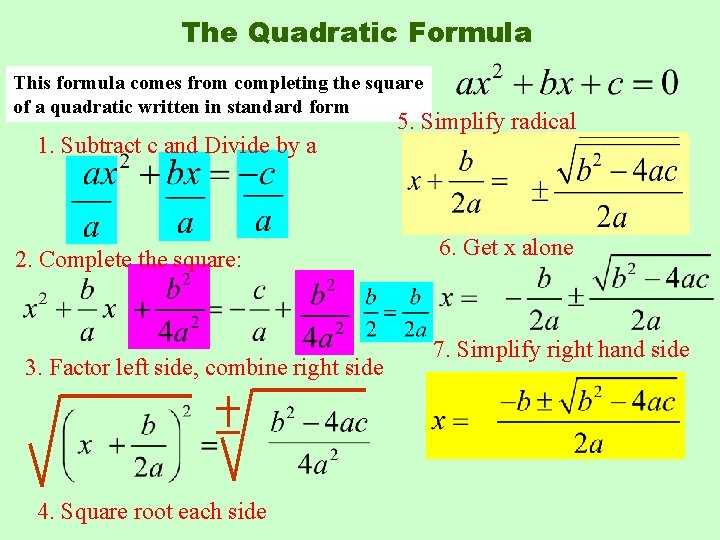The Quadratic Formula This formula comes from completing the square of a quadratic written in standard form 1. Subtract c and Divide by a 2. Complete the square: 3. Factor left side, combine right side 4. Square root each side 5. Simplify radical 6. Get x alone 7. Simplify right hand side“x equals opposite b plus or minus square root of b squared minus 4 ac all over 2 a”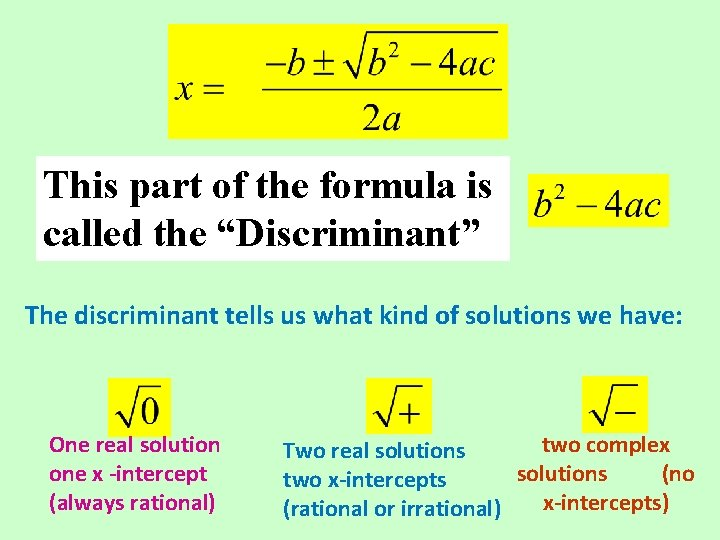This part of the formula is called the “Discriminant” The discriminant tells us what kind of solutions we have: One real solution one x -intercept (always rational) two complex Two real solutions (no two x-intercepts) (rational or irrational)The Quadratic Formula Solve the equation 1. Identify a, b, c 6. Simplify a=4 b= 2 c=5 2. Plug into the formula 2 (2)2 (4) 5. Simplify Notice the solutions are complex! =4 • 19 7. Simplify radical (4) (5) 8. Simplify final answer, if possible Meaning: 0 xintercepts, 2 complex solutions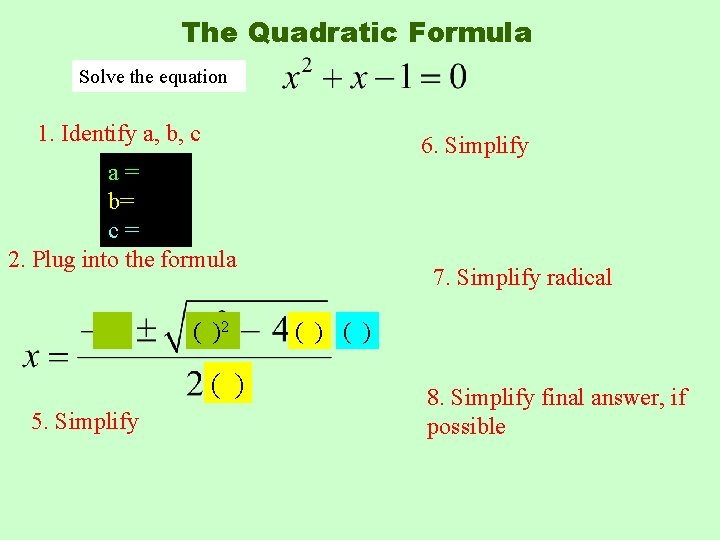The Quadratic Formula Solve the equation 1. Identify a, b, c 6. Simplify a= b= c= 2. Plug into the formula ( )2 ( ) 5. Simplify 7. Simplify radical ( ) 8. Simplify final answer, if possible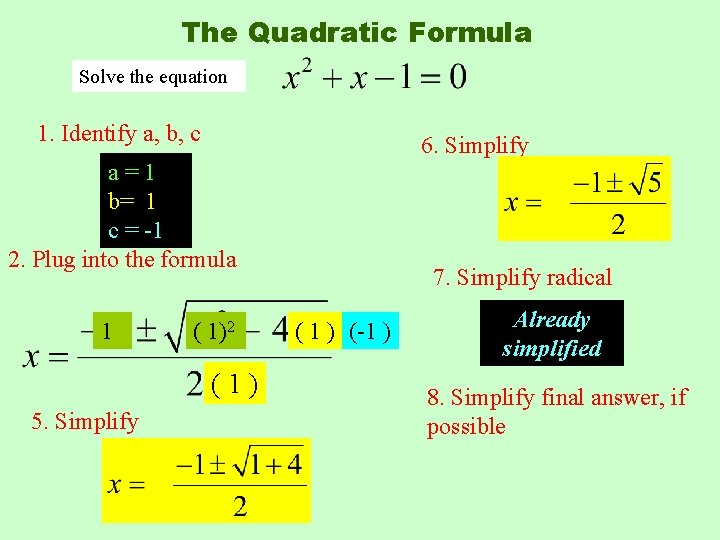The Quadratic Formula Solve the equation 1. Identify a, b, c 6. Simplify a=1 b= 1 c = -1 2. Plug into the formula 1 ( 1)2 (1) 5. Simplify 7. Simplify radical ( 1 ) (-1 ) Already simplified 8. Simplify final answer, if possibleAnother example Solve the equation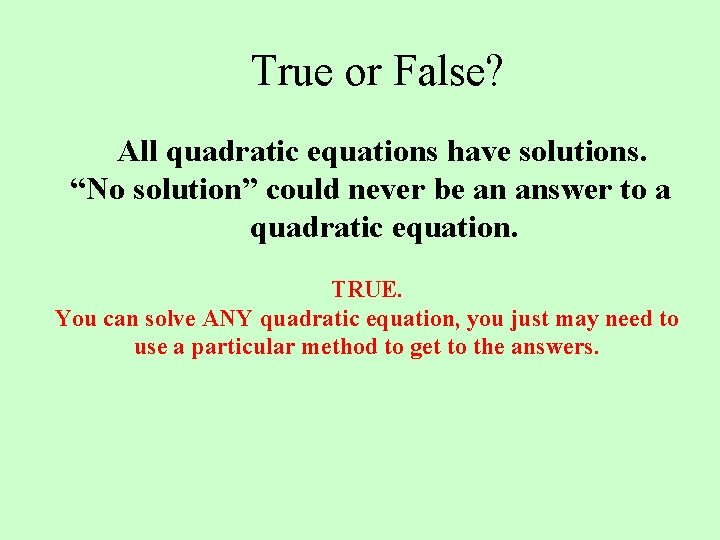True or False? All quadratic equations have solutions. “No solution” could never be an answer to a quadratic equation. TRUE. You can solve ANY quadratic equation, you just may need to use a particular method to get to the answers.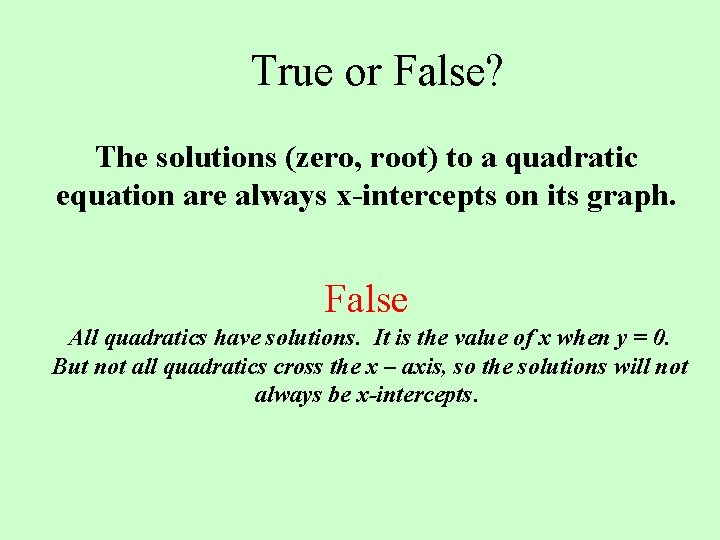True or False? The solutions (zero, root) to a quadratic equation are always x-intercepts on its graph. False All quadratics have solutions. It is the value of x when y = 0. But not all quadratics cross the x – axis, so the solutions will not always be x-intercepts.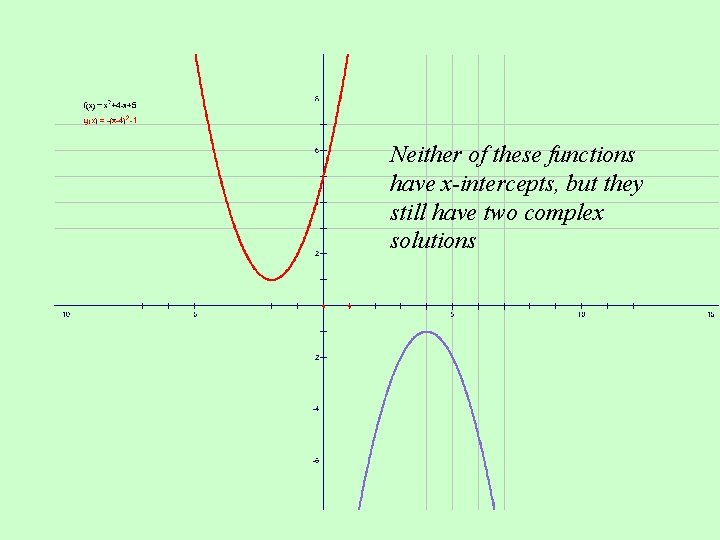Neither of these functions have x-intercepts, but they still have two complex solutionsTrue or False? All quadratics equations can be factored. False Here are just a few examples of quadratics that cannot be factored: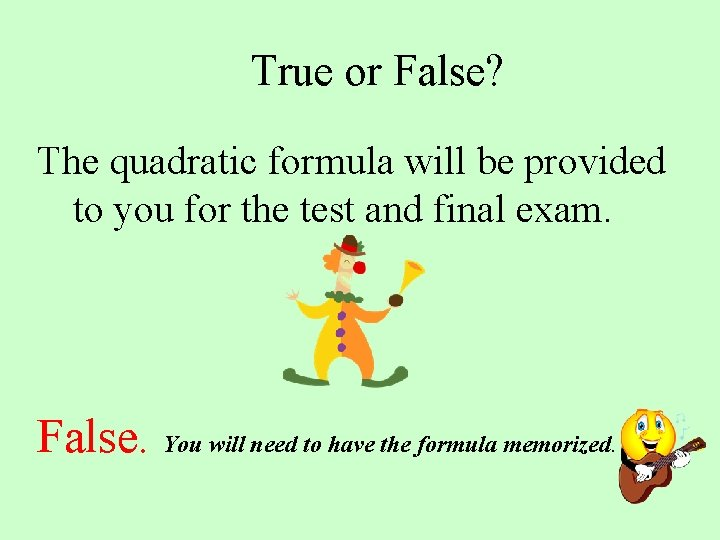True or False? The quadratic formula will be provided to you for the test and final exam. False. You will need to have the formula memorized.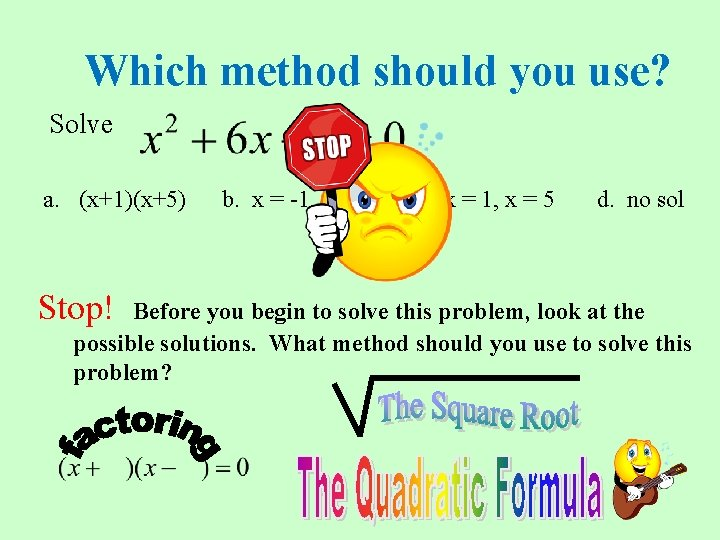Which method should you use? Solve a. (x+1)(x+5) Stop! b. x = -1, x = -5 c. x = 1, x = 5 d. no sol Before you begin to solve this problem, look at the possible solutions. What method should you use to solve this problem?Which method should you use? Solve a. b. c. no sol because you cannot factor it Stop! Before you begin to solve this problem, look at the possible solutions. What method should you use to solve this problem?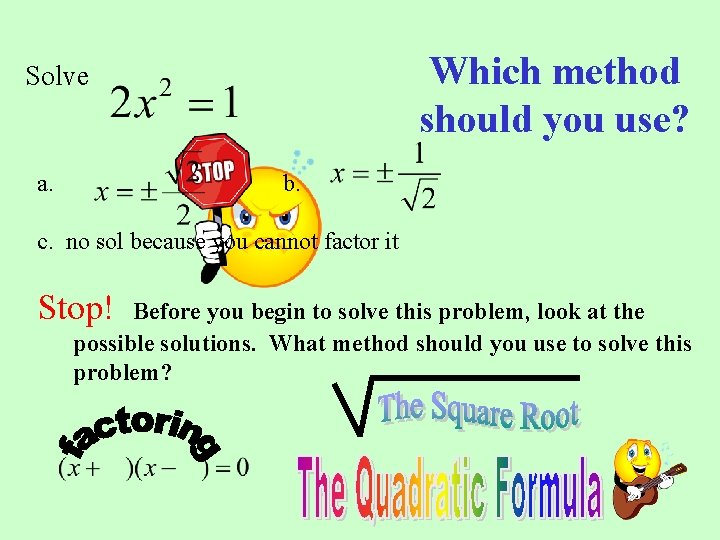Which method should you use? Solve a. b. c. no sol because you cannot factor it Stop! Before you begin to solve this problem, look at the possible solutions. What method should you use to solve this problem?Which method should you use? Solve a. b. c. d. Stop! Before you begin to solve this problem, look at the possible solutions. What method should you use to solve this problem?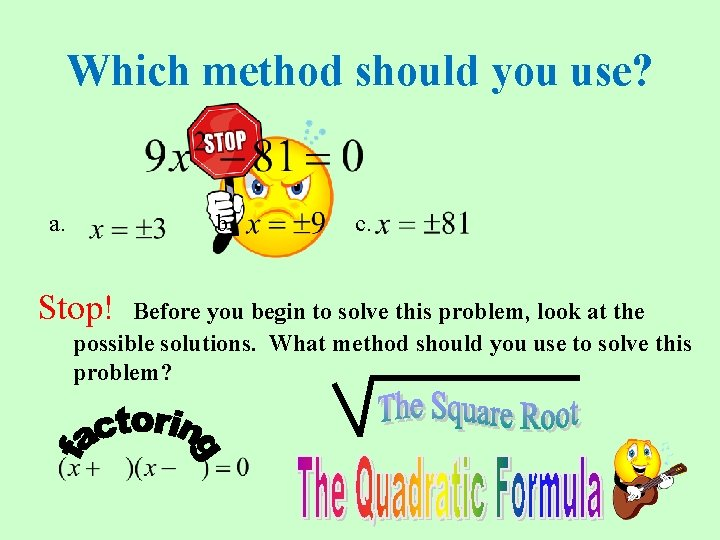Which method should you use? a. b. Stop! c. Before you begin to solve this problem, look at the possible solutions. What method should you use to solve this problem?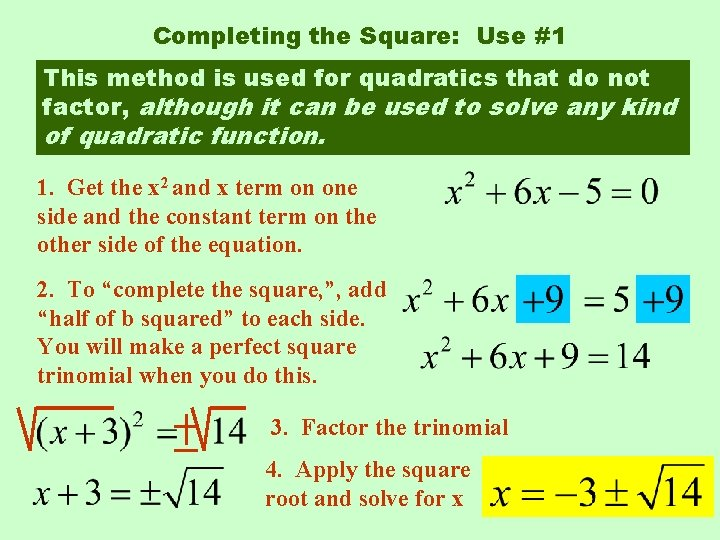Completing the Square: Use #1 This method is used for quadratics that do not factor, although it can be used to solve any kind of quadratic function. 1. Get the x 2 and x term on one side and the constant term on the other side of the equation. 2. To “complete the square, ”, add “half of b squared” to each side. You will make a perfect square trinomial when you do this. 3. Factor the trinomial 4. Apply the square root and solve for x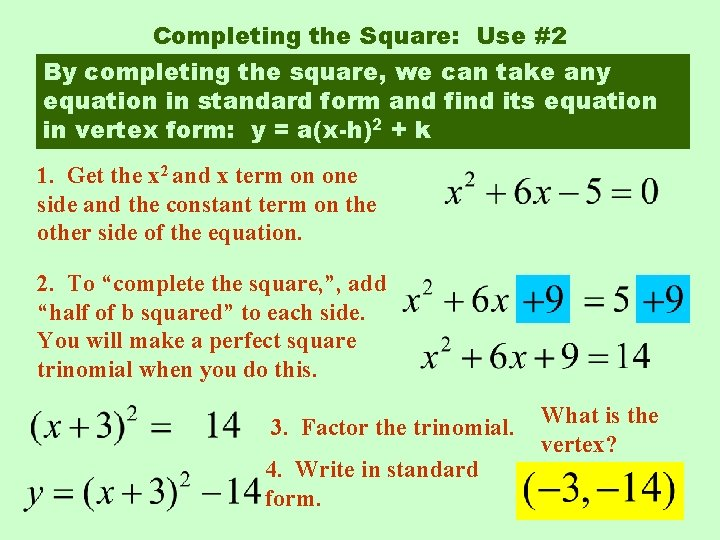Completing the Square: Use #2 By completing the square, we can take any equation in standard form and find its equation in vertex form: y = a(x-h)2 + k 1. Get the x 2 and x term on one side and the constant term on the other side of the equation. 2. To “complete the square, ”, add “half of b squared” to each side. You will make a perfect square trinomial when you do this. 3. Factor the trinomial. 4. Write in standard form. What is the vertex?